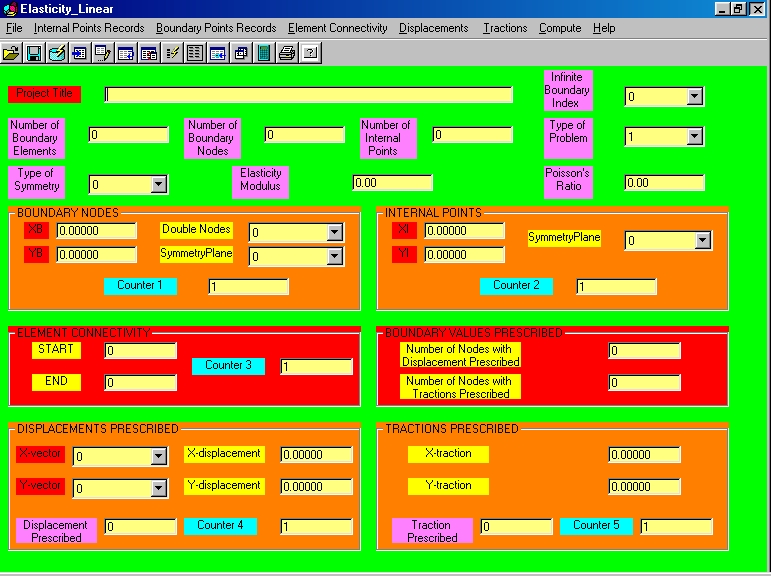Program 6: Two-dimensional Elasticity with Linear Elements (Elasticity_Linear)A. GENERAL INFORMATION

1. The current program is a result of the S.I.O.M. ( Singular Integral Operators Method ) for the  solution  of  two-
dimensional elasticity problems for isotropic solids with linear elements.

2. The capabilities of this elasticity computer program is the computation of the dispacements, the tractions and the stresses on the boundary and at any internal points.

3. The current program can be used for the solution of elasticity problems with several surfaces,  like  bodies  with
holes, etc.

4. Moreover this program can be used for both finite and infinite boundaries.

B. SPECIAL INFORMATION

1. INFINITE BOUNDARY INDEX: 1 means that there a boundary at infinity, 0 if the body is bounded.

2. INDEX FOR TYPE OF PROBLEM: 1 for plane stress, 2 for plane strain.

3. INDEX FOR SYMMETRY: 0 for no-symmetry , 1 for plane of symmetry normal to Y, 2 for plane of symmetry normal to X , 3 for two planes of symmetry normal to X and Y.

4. DOUBLE NODE: 0 for no double node ( = number of node with the same coordinates); 1 for double node.

5. SYMMETRY PLANE INDEX: Indicates if the boundary node or internal point is located on any symmetry plane: 0 if not, 1 for plane normal to Y, 2 for plane normal to X, 3 if located at the intersection of the symmetry planes.

6. X -,Y -VECTOR FOR PRESCRIBED DISPLACEMENTS: 0 if traction is prescribed, 1 if displacement is prescribed.

C. DATA ENTRY - OUTPUT RESULTS

1. The program opens a file *.dat (data entry). This file includes all the requested data.

2. The results of the program are given by a file *.res (output results).

3. Both data entry (file *.dat) and output results (file *.res) are printed by the program.

D. KEYS OF THE PROGRAM

The Records are referred to:

a. Internal Points (Coordinates).

b. Boundary Points (Coordinates and Boundary Conditions).

c. Element Connectivity.

d. Displacements Prescribed (Boundary Conditions).

e. Tractions Prescribed (Boundary Conditions).

The keys are:

1. Add Records: Records are added by placing in Counter a number 1,2,3, ...etc.and then by pressing key "Add".

2. Edit Records: Records are edited by placing in Counter a number 1,2,3, ...etc and then by pressing key "Edit".

3. Erase Records: In order to erase a specific Coordinate, write in Counter its corresponding number and then press "Erase".

4. Insert Records: In order to insert a specific Coordinate between two other existing coordinates, write in Counter its corresponding number and then press "Insert".